• 在一些公司的面试中可能会有些面试官会出一些奇奇怪怪的问题，比如怎么样计算1加到100的和要求使用加法，减法，循环和if判断，再比如怎么样不用第三个变量交换两个变量的等问题。这里我们先分析下怎么样不用...
前言

在一些公司的面试中可能会有些面试官会出一些奇奇怪怪的问题，比如怎么样计算1加到100的和要求不能使用加法，减法，循环和if判断，再比如怎么样不用第三个变量来交换两个变量的值等问题。这里我们先分析下怎么样不用第三个变量来交换两个变量的值。

正文

通常情况下我们会用第三个变量来进行两个变量的交换，比如在冒泡排序中会经常用到。那么怎么样不用第三个变量而只通过两个变量本身来进行交换呢？

swap(a,b); //C++自带的一个交换函数

swap()这个函数在C++中可以直接用来交换两个变量，使用很方便，但是如果不能用自带函数呢？

a=a+b;//a的值变成了a+b的和
b=a-b;//相当于a+b-b，结果就是原来a的值赋值给了b
a=a-b;//相当于a+b-a，结果就是把原来的b的值赋值给了a

这种办法很巧妙的对两个变量进行了交换，而不用第三个变量，但是需要注意的是变量的范围，比如这两个变量都是int，而int的取值范围是~，如果a这个时候刚好是，那么再加上b是不是就超过了int的范围了？是不是就无法完成了交换了？不会。因为当cpu计算完a+b的时候这个结果会缓存在cache中，当下次要继续用这个结果的时候会直接从cache中读取，而不会再次从内存中读取，这样的读取方式能很大程度上提高程序的运行速度。

展开全文C++
• 方法1：实现个变量值交换不使用第三个变量 我们可以通过数学运算中的差值来实现交换   int a=10,b=20;     a=a+b;     b=a-b;     a=a-b;     交换成功，运行结果如下图 ...
方法1：实现两个变量值交换，不使用第三个变量
我们可以通过数学运算中的差值来实现交换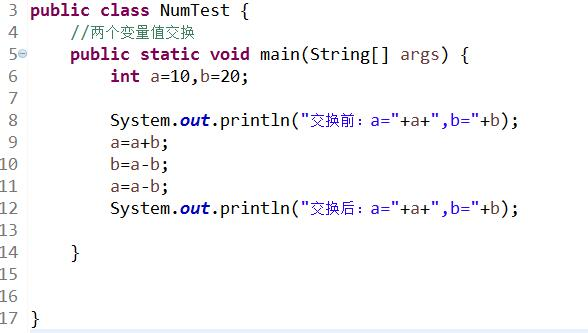int a=10,b=20;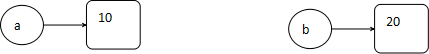a=a+b;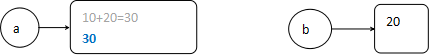b=a-b;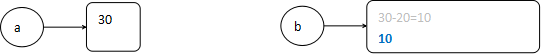a=a-b;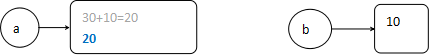交换成功，运行结果如下图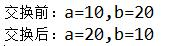方法2：实现两个变量值交换，使用第三个变量
我们将a看做咖啡，b看做可乐，当我们要讲a,b两个杯子的饮品交换时，一般会借助一个空杯子t来实现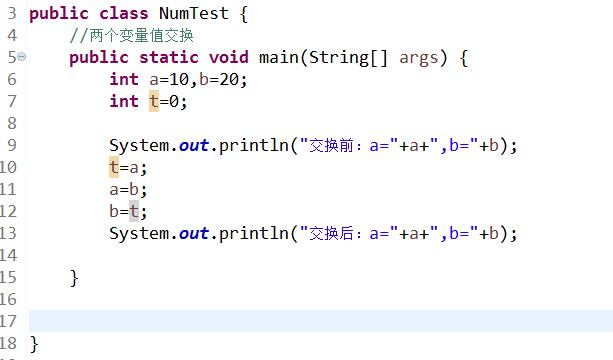int a=10,b=20;int t=0;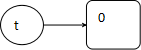因此第9行打印交换前的结果是: a=10,b=20
t=a;  //这里我们可以看做是将咖啡a倒入了空瓶t中，此时a的存储空间被释放了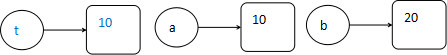a=b;  //这里我们可以看做是将可乐b倒入了被释放了空间的a中，此时b的存储空间被释放了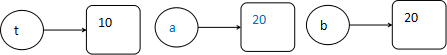b=t; //这里我们可以看做是将t杯中的咖啡倒入了被释放了空间的b中，此时a，b被交换了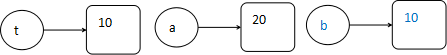所以第13行打印交换后: a=20,b=10展开全文• 不使用第三个变量怎么交换两个变量的 异或运算交换 二进制数字异或 ：相同为零(假) ，不同为一(真) 如图，完成n1和n2的交换 1.2.4.2 相加再相减交换 如图n1,n2进行交换 ...
不使用第三个变量怎么交换两个变量的值
异或运算交换
			二进制数字异或 ：相同为零(假) ，不同为一(真)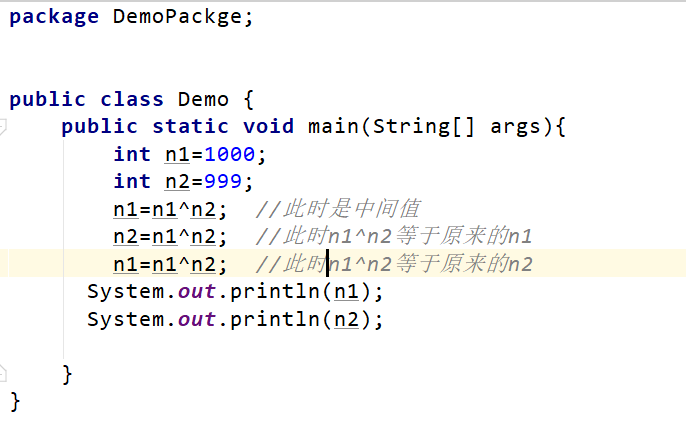如图，完成n1和n2的交换
1.2.4.2  相加再相减交换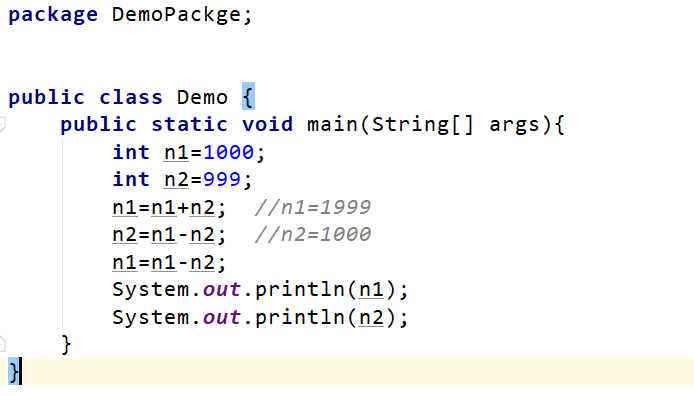如图n1,n2值进行交换


展开全文Java 题目
• C语言不使用第三个变量交换两个变量的 #include<stdio.h> int main(int argc, char argv[]) { int a=3,b=4,c=3,d=4; printf("交换前:a=%d,b=%d\n",a,b); a^=b; b^=a; a^=b; printf("交换后:a=%d,b=%d\n",a,b...
C语言不使用第三个变量交换两个变量的值
#include<stdio.h>
int main(int argc, char argv[])
{
int a=3,b=4,c=3,d=4;
printf("交换前:a=%d,b=%d\n",a,b);
a^=b;
b^=a;
a^=b;
printf("交换后:a=%d,b=%d\n",a,b);
printf("交换前:c=%d,d=%d\n",c,d);
c=c+d;
d=c-d;
c=c-d;
printf("交换后:c=%d,d=%d\n",c,d);
return 0;
}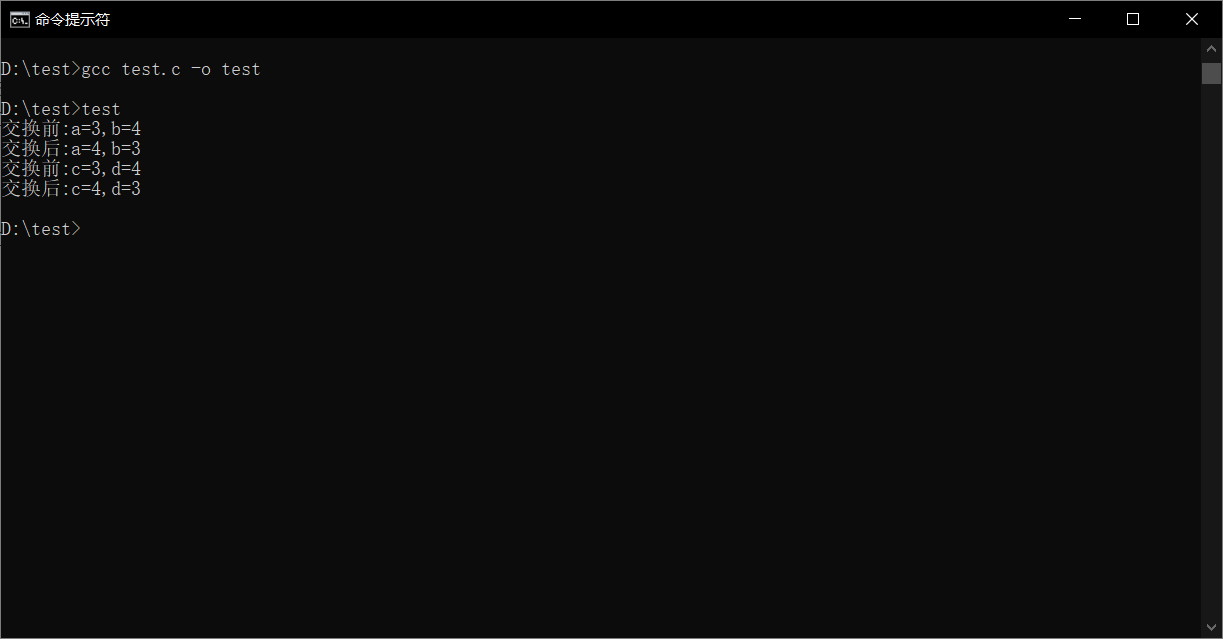展开全文• b=12,要求在不使用第三个变量的情况下相互交换值。 下面是个人愚见，欢迎吐槽~~ $a = 10;$b = 12; //运用算法 $a =$a + $b;$b = $a-$b; $a =$a-$b; //字符串截取法$len_a = strlen($a);$a = $a .$b; $b ... • 下面给出几种不使用第三个变量的办法： 1.利用运算交换两个变量 a = b - a; b = b - a; a = b + a; 2.位运算 通过异或运算来交换 a=a^b; b=a^b; a=a^b; 3.交换指针地址 转载于:... • 一、引入 通常我们的做法是（尤其是在学习阶段）：定义一新的变量，借助它完成交换。...二、四种借助第三变量交换方法 上面的算法最大的缺点就是需要借助一临时变量。那么借助临时变量可以实现交换前端 • Python中不使用第三个变量交换两个数值变量的 假设已知两个变量: x, y = 2, 3 交换两个变量的 常规思路：新建一个变量作为临时容器暂存数据，然后进行交换 temp = 0 temp = x x = y y = temp print(x, y)... • 两个整形变量互换（不增加第三变量） 问题描述 定义整型变量 a、b，写出将 a、b 两个变量进行互换的程序 ...定义两个整形变量，如a和b，并赋值，在不使用第三个变量的条件下，将两个变量的互换。 ...java 算法 • 通常我们的做法是（尤其是在学习阶段）：定义一新的变量，借助它完成交换。代码如下： int a,b; a=10; b=15; int t; t=a; a=b; b=t; 这种算法易于理解，特别适合帮助初学者了解计算机程序的特点，是赋值语句... •C • 以上这篇Python3之不使用第三方变量,实现交换两个变量就是小编分享给大家的全部内容了，希望能给大家一个参考，也希望大家多多支持软件开发网。 您可能感兴趣的文章:python交换两个变量方法对Python中实现... • 用PHP写一段代码，实现不使用第3个变量交换$a、$b的，$a、$b的初始自己定。$a="hello"; $b="world"; 在Vb语言中，有直接的函数实现两个变量的互换，而在PHP中，确实还不是那么容易。 这里介绍种实现。... • 交换两个变量值，没有要求的时候原理为：定义一个中间变量，将a的赋于中间变量，b的赋于a，最后将中间变量的赋于b即可 但是如果要求使用第三变量来达到交换两个变量值的目的呢？ 程序运行结果如下图： • Python3之不使用第三方变量,实现交换两个变量method 1：a，b = b，amethod 2：a = a+bb = a-ba = a-b以上这篇Python3之不使用第三方变量,实现交换两个变量就是小编分享给大家的全部内容了，希望能给大家一个... • 交换两个变量的不使用第三个变量的四种法方 通常我们的做法是（尤其是在学习阶段）：定义一个新的变量，借助它完成交换。代码如下： int a,b; a=10; b=15; int t; t=a; a=b; b=t; 这种算法易于理解，特别适合... • 在一些嵌入式开发中，有可能内存空间不够，...交换两个变量的的时候，常常不使用第三个变量，这样可能节约一个寄存器的使用。具体方法有两种方法一：a=1；b=2；a=a+b;b=a-b;a=a-b;方法二：a=1;b=2;a=a^b;b=a^b;a=a^b;嵌入式 • 在这里提供2种需要创建第三个变量就能交换2个变量的的方法。 这里以快排中，交换已知2个下边对应的元素作为例子 先来看需要创建第三个变量的方法： public static void swap(int[] arr, int i, int j) { ... • 第二种方法就是不使用第三个变量的情况下交换两个变量。下面我们就通过具体的代码示例，给大家介绍PHP交换两个变量的方法。首先大家要知道交换两个变量就是指相互交换变量的。通常，这是通过内存中的数据完成... • 交换的为数字时： /** * 双方变量为数字时，可用交换方法五 * 使用加减运算符，相当于数学运算了^_^ ... // a变量原始 ... echo '交换之前$a 的：'.$a.',$b 的：'.$b,''; // 输出原始 • “PHP不用第三个变量交换两个变量的”这个题看到过好多次了，看来面试确实喜欢考这道题。今天，对于这个题目，我自己总结了几种方法，可能全，大家来互相补充。有些仅适用于字符串，方法二和方法五比较不错。... • /* 交换两个变量的不使用第三个变量 */ #include using namespace std; void Swap1(int& a, int& b) { if(a ) { a=b-a; b=b-a; a=b+a; } else { b = a - b; a = a - b; b... • 首先是使用 第三方变量交换两个变量； static void Main(string[] args) { int a = 3; int b = 5; JH(ref a, ref b); Console.WriteLine("a的是" + a + "b的是"... • 适用第三个变量交换两个变量之间的值得方法有三种： 1:$a = 3; $b = 4;$a = $a +$b; $b =$a - $b;$a = $a -$b; 2: $a = 3;$b = 4; $a =$a ^ $b;$b = $a ^$b; $a =$a ^ $b; 3:$aPHP...